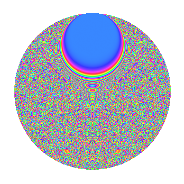# Properties

 Label 3004.2.rLevel 3004 Weight 2 Character orbit r Rep. character $$\chi_{3004}(195,\cdot)$$ Character field $$\Q(\zeta_{50})$$ Dimension 7480 Sturm bound 752

# Related objects

## Defining parameters

 Level: $$N$$ = $$3004 = 2^{2} \cdot 751$$ Weight: $$k$$ = $$2$$ Character orbit: $$[\chi]$$ = 3004.r (of order $$50$$ and degree $$20$$) Character conductor: $$\operatorname{cond}(\chi)$$ = $$3004$$ Character field: $$\Q(\zeta_{50})$$ Sturm bound: $$752$$

## Dimensions

The following table gives the dimensions of various subspaces of $$M_{2}(3004, [\chi])$$.

Total New Old
Modular forms 7560 7560 0
Cusp forms 7480 7480 0
Eisenstein series 80 80 0

## Trace form

 $$7480q - 40q^{2} - 40q^{4} - 40q^{5} - 30q^{6} - 40q^{8} - 40q^{9} + O(q^{10})$$ $$7480q - 40q^{2} - 40q^{4} - 40q^{5} - 30q^{6} - 40q^{8} - 40q^{9} - 10q^{10} - 40q^{12} - 120q^{13} - 20q^{14} - 80q^{16} - 40q^{17} - 20q^{18} - 40q^{21} + 10q^{22} - 10q^{24} - 60q^{25} - 15q^{26} - 45q^{28} - 40q^{29} + 20q^{30} - 80q^{32} - 10q^{33} - 25q^{34} + 20q^{36} - 100q^{37} + 15q^{38} - 75q^{40} - 50q^{41} - 120q^{42} + 20q^{44} + 20q^{45} + 5q^{46} - 25q^{48} - 40q^{49} - 20q^{50} + 65q^{52} - 30q^{53} - 20q^{54} + 160q^{56} - 240q^{57} - 65q^{58} + 105q^{60} - 30q^{61} + 5q^{62} - 160q^{64} + 30q^{66} - 20q^{68} - 100q^{69} - 25q^{70} - 40q^{72} - 25q^{74} + 40q^{76} - 10q^{77} - 40q^{80} - 40q^{81} - 25q^{82} - 20q^{84} - 50q^{85} - 160q^{86} + 20q^{88} - 60q^{89} - 20q^{90} - 400q^{92} - 100q^{93} - 20q^{94} - 35q^{96} - 20q^{97} - 105q^{98} + O(q^{100})$$

## Decomposition of $$S_{2}^{\mathrm{new}}(3004, [\chi])$$ into irreducible Hecke orbits

The newforms in this space have not yet been added to the LMFDB.